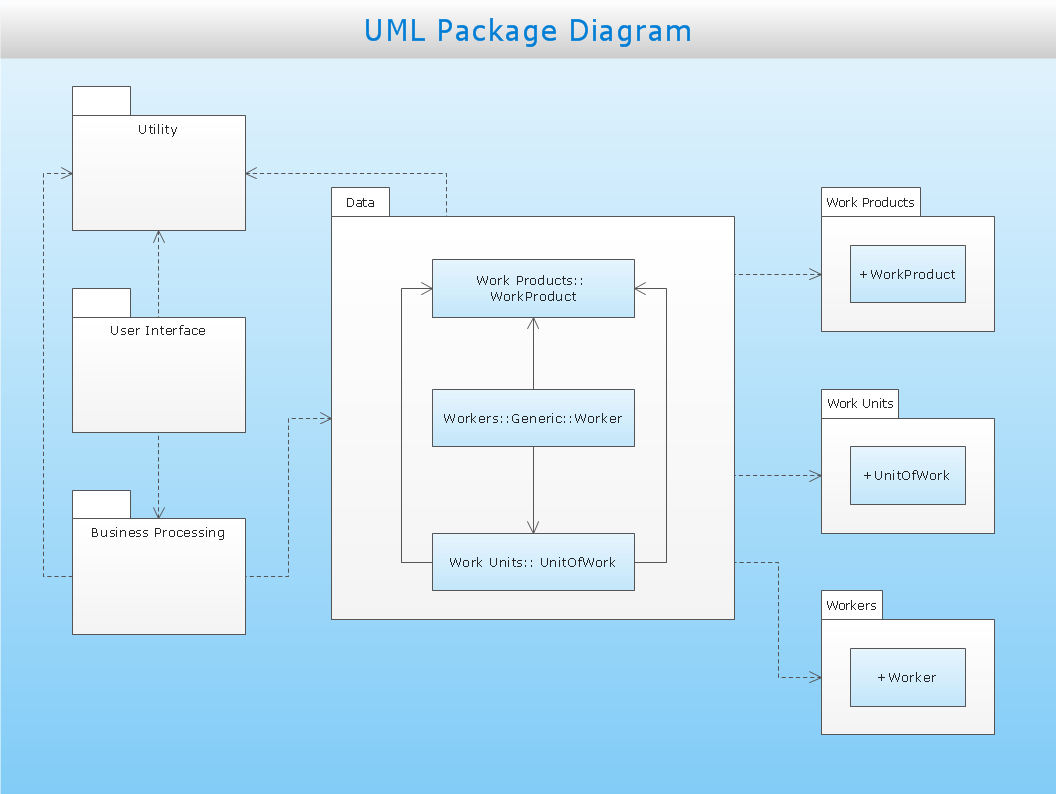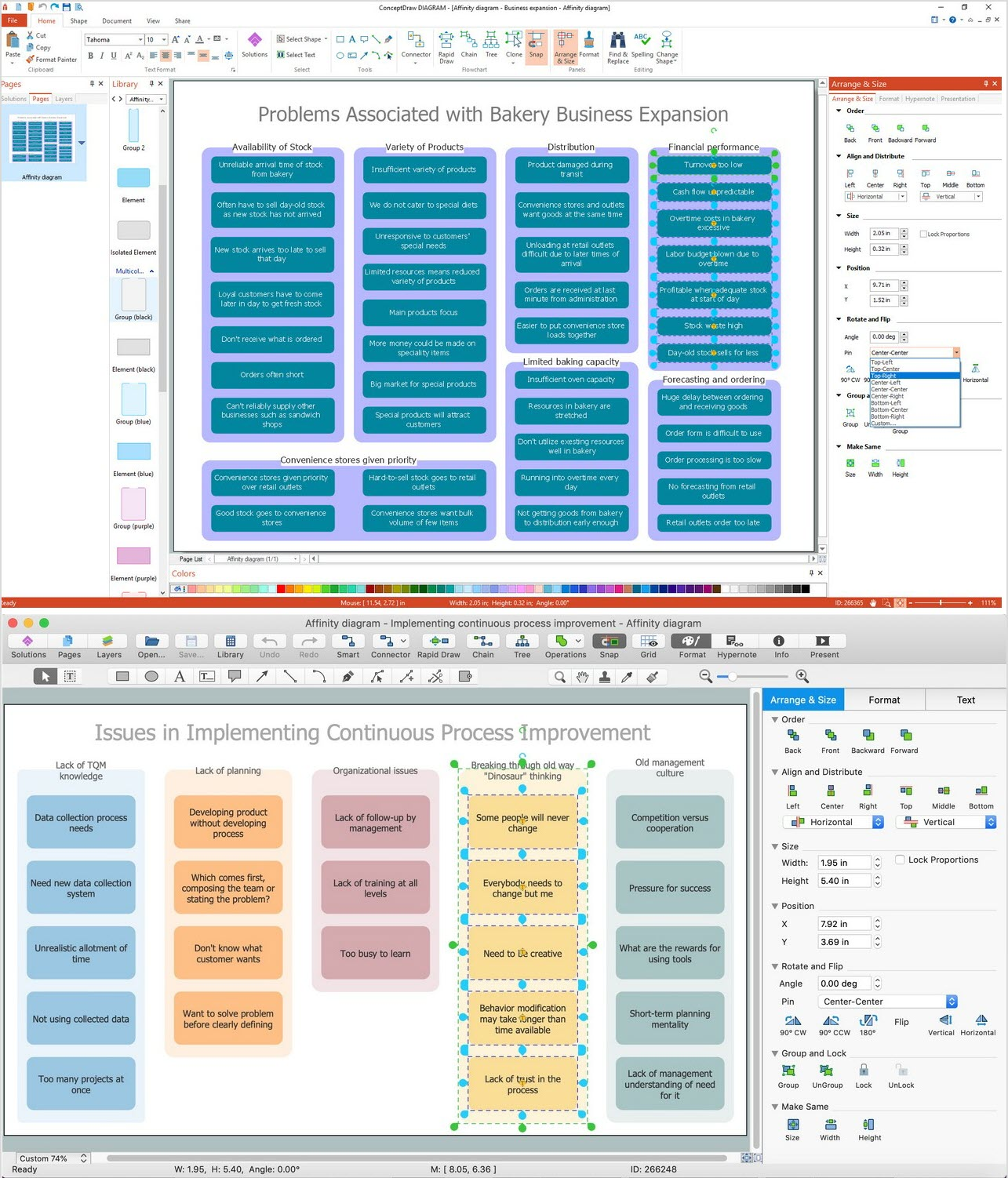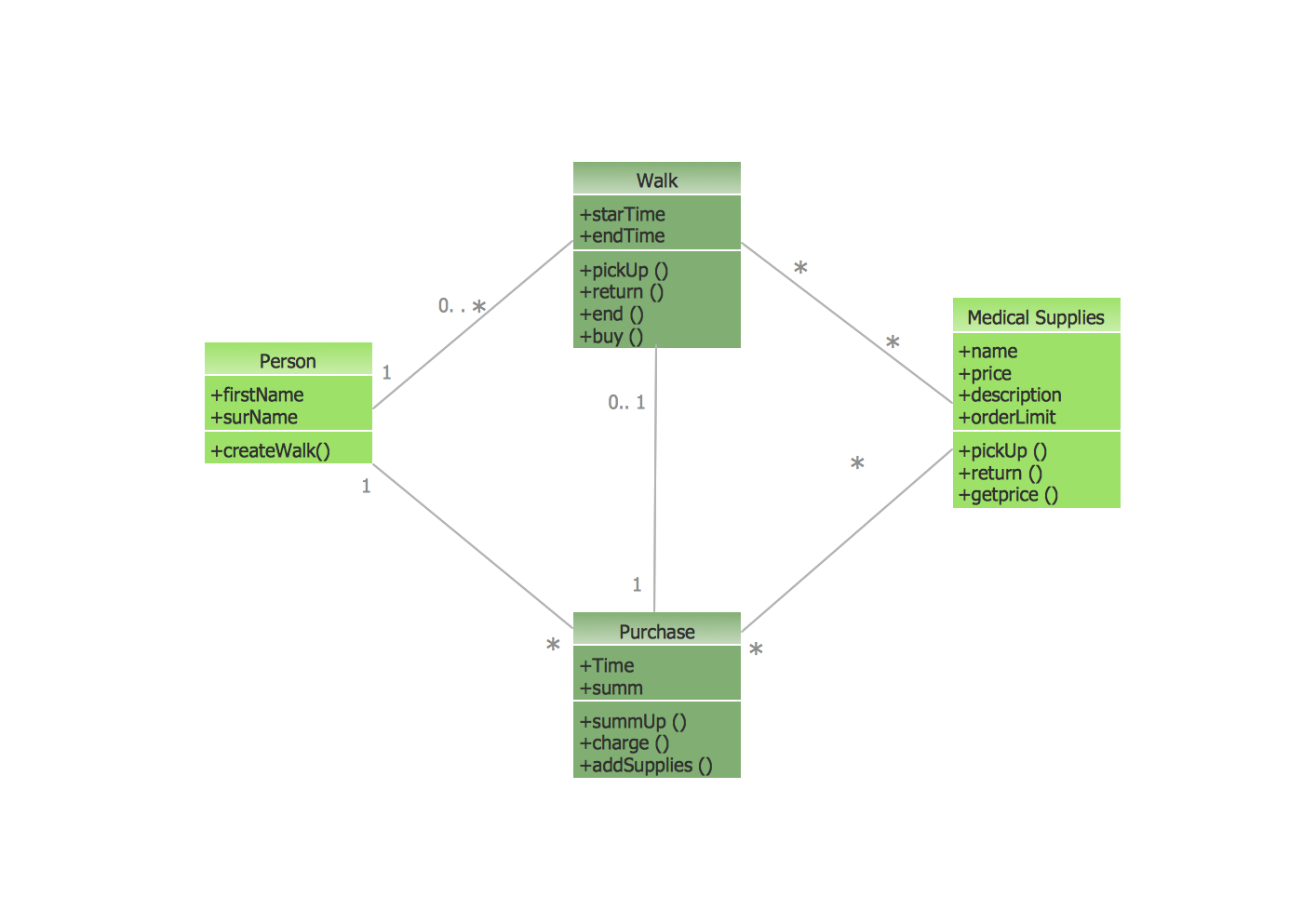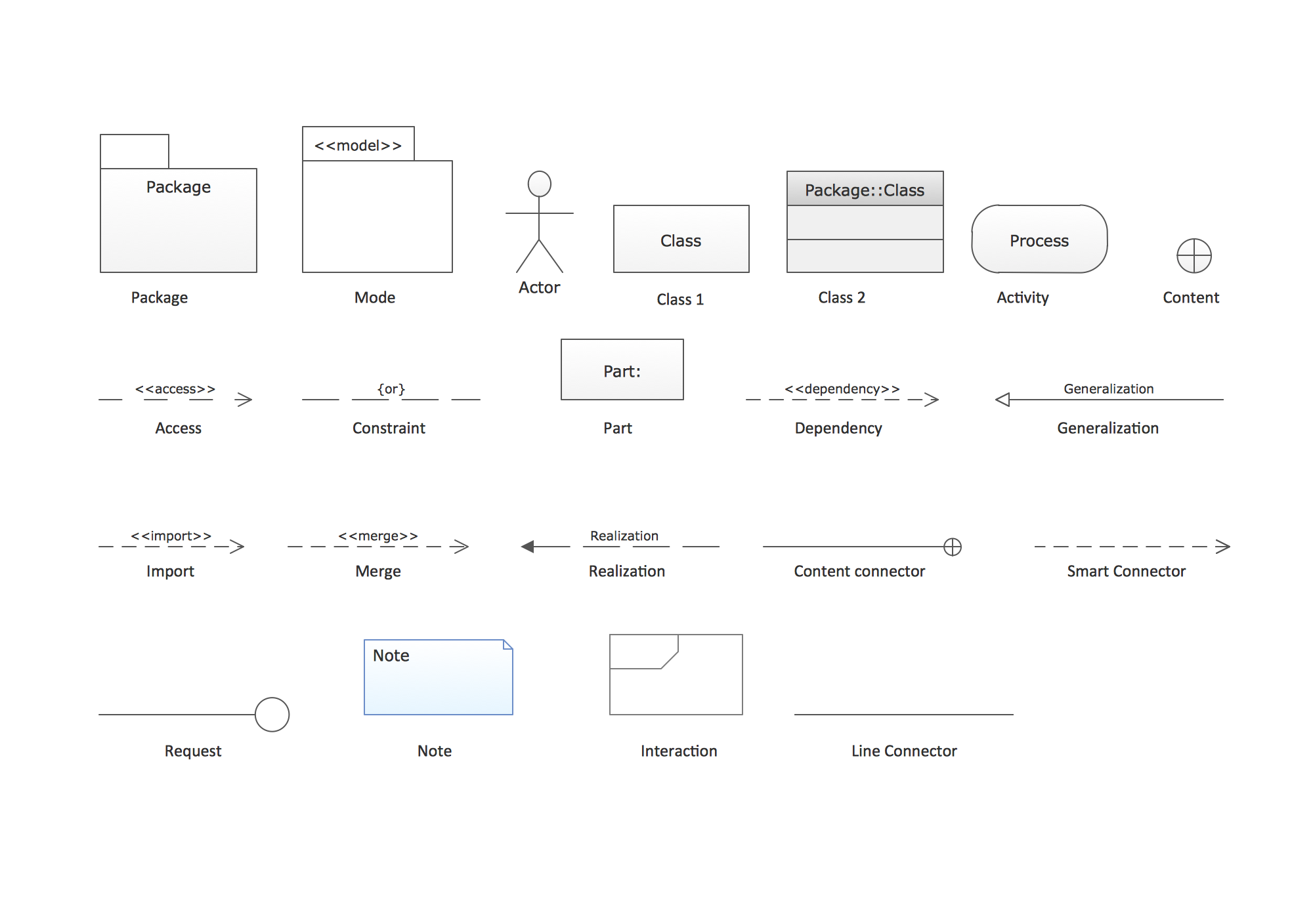ConceptDraw
DIAGRAM 15

# UML Package Diagram

## How To draw UML Package Diagrams

UML Package Diagram depicts the dependencies between the packages that make up a model. There are two special types of dependencies defined between packages:

• package import
• package merge
UML Package Diagram illustrates the functionality of a software system.
ConceptDraw has three examples that will help you to start using software for drawing UML Package Diagrams. You can use the appropriate stencils of UML notation for drawing your own UML Package Diagram.This UML package diagram sample is created using ConceptDraw DIAGRAM diagramming and vector drawing software enhanced with Rapid UML solution from ConceptDraw Solution Park.

Rapid UML solution provides templates, examples and libraries of stencils for quick and easy drawing all the types of system and software engineering diagrams according to UML 2.4 notation.

Use ConceptDraw DIAGRAM with UML package diagram templates, samples and stencil library from Rapid UML solution to show the dependencies between the packages that make up your system model.

### Activity on Node Network Diagramming Tool →

Activity on Node Network Diagramming Tool - Activity Network and Project Evaluation and Review Technique, or PERT, charts are a way of documenting and analyzing the tasks in a project. This sample shows the Activity on node network diagramming method. It was created in ConceptDraw DIAGRAM diagramming and vector drawing software using the Seven Management and Planning Tools solution from the Management area of ConceptDraw Solution Park.Picture: Activity on Node Network Diagramming Tool
Related Solution:

### UML Notation →

There are many ways to track the system in a critical situation. To model a system behavior, uml notation is widely used. Usually, an UML diagram consists of elements such as actor and a case. This diagram represents the structure of UML notations. Unified Modeling Language (UML) is used in software engineering to depict graphically the software modeling process. UM Language uses graphic notations for developing models of object-oriented systems. These notations displays requirements, sub-systems, logical and physical elements, etc. We created this diagram using ConceptDraw DIAGRAM reinforced with Rapid UML solution. It can be helpful for students on software engineering, when learning UML.Picture: UML Notation
Related Solution:

### UML Package Diagram. Design Elements →

UML Package Diagram illustrates the functionality of a software system. ConceptDraw has 393 vector stencils in the 13 libraries that helps you to start using software for designing your own UML Diagrams. You can use the appropriate stencils of UML notation from UML Package library.Picture: UML Package Diagram. Design Elements
Related Solution:
ConceptDraw
DIAGRAM 15